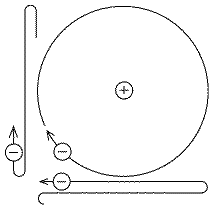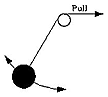(Q-5) The Atomic Nucleus and Bohr's Early Model of the AtomIndexS-2.Solar LayersS-3.The Magnetic SunS-3A. Interplanetary
Magnetic FieldsS-4. Colors of SunlightS-4A.Color Expts.S-5.Waves & Photons

Optional: Quantum PhysicsQ1.Quantum PhysicsQ2. AtomsQ3. Energy LevelsQ4. Radiation from
Hot ObjectsQ5.The Atomic Nucleus
and Bohr's ModelQ6. Expansion of
Bohr's ModelQ7.Wave MechanicsQ8. Tunneling
--------------------------S-6.The X-ray SunS-7.The Sun's EnergyS-7A. The Black Hole at
our Galactic CenterLS-7A. Discovery
of Atoms and NucleiS-8.Nuclear PowerS-9.Nuclear Weapons

The Atomic Nucleus

Planck's law described the way hot matter in bulk radiated energy. To gain information about atoms themselves, however, one needs to understand the intricate structure of energy levels, as deduced from atomic spectra, emitted by hot gases whose atoms act independently. What were atoms like, and what determined those levels?

J.J. Thomson, discoverer of the electron, thought the atom may consist of a positively charged mass, inside which negative electrons were embedded ("like raisins in a pudding"), and others speculated on details of such a model. Viewed from the outside, the electric forces from opposing positive and negative charges would balance each other, so from a distance any atom would appear electrically neutral. However, electrons were expected to oscillate around their equilibrium positions, and to radiate with the characteristic frequencies of their oscillation, in accordance with Hertz'es theory. Einstein's formula

E = hν

then suggested a link between the frequencies of oscillation and the energies radiated.

This neat picture was upset by a remarkable experiment performed in Manchester in 1909 by Ernest Rutherford, originally from New Zealand. It was known by then that heavy radioactive elements emitted "alpha particles" (α particles), fast ions of helium lacking both their electrons. That gave them a positive charge of +2e, twice the electron's charge of –e.

Rutherford knew how to detect such ions individually. In total darkness, if they were allowed to hit a specially prepared fluorescent screen, the flash they produced was just bright enough to be seen by the dark-adapted eye, observing a small part of the screen through a microscope. The α particles were found to have enough energy to penetrate a thin gold foil, and Rutherford therefore set his assistant Hans Geiger (later assisted by Ernest Marsden) to examine how passage through such a foil affected their motion.

With an atom resembling the one proposed by J.J. Thomson, the ions would feel no electric force while outside the gold atom, where effects of positive and negative charges cancelled. If they sliced through the edge of the atom, calculations suggested that the distributed positive electric charge would bend their path slightly.

What Geiger and Marsden saw, however, was quite different. Most α particles indeed went through the foil with only small deflections. But a few were scattered by large angles, in a way which could only be explained by assuming that the positive charge of the gold atom and most of its mass formed a tiny point-like concentration. Only then was the bombarding particle able to approach it so close that its electric repulsion could deflect it by a big angle or even turn it back. Rutherford named this concentration the atomic nucleus. The electrons stayed far outside, like planets orbiting far from the Sun, a view indeed suggested by Hantaro Nagaoka in Japan.It looked like a miniature planetary system, with the attraction between positive nucleus and negative electrons filling the place of gravity--an attraction which, like gravity, decreased like the inverse square of the distance R, like 1/R2. An electron held in this manner should obey Kepler's laws, like a planet attracted by gravity, and move in a circle or ellipse. Its motion can then be viewed as the sum-total of two perpendicular oscillations, each reaching its greatest extent at the moment the other one passes the middle point (just consider the "shadows" of the motion on the x and y axes, shown in the drawing !).

Each of these two (independent) motions is equivalent to an electric charge oscillating back and forth around an equilibrium point. By the laws of Maxwell and Hertz, however, such an electron is expected to emit electromagnetic radiation, which would reduce its energy. Because of this, unlike planets of the solar system, the electrons would quickly radiate away their energy and spiral inwards, until they reached the nucleus

Atoms, however, are (fortunately!) stable. How come?

Bohr's Model of the Atom

The results of Balmer and Ritz seemed to tell that atoms had stable energy levels, and Einstein's formula suggested that energy at the atomic level seemed to be related to the Planck's constant h, measured in joule-seconds.

One quantity measured in joule-seconds was angular momentum.     That is a mechanical property (just as energy, say) of any rotating object or collection of objects--even of the Earth, Sun or the solar system. It measures the total "amount of rotation"--adjusted for the mass involved, its average distance from the axis of rotation, and of course, its rate of rotation. It is a vector quantity, its direction following the rotation axis.

The rotations of Earth and Sun, and orbital motions of planets and moons, do involve angular momentum. A young Danish physicist named Niels Bohr, who arrived in England in 1912 and ended up working with Rutherford at the University of Cambridge, looked into the angular momentum of an electron orbiting a nucleus. It was guessed by then that as dimensions of a motion shrank to the atomic scale, Newton's laws were gradually modified, allowing electrons to have orbits where (for some yet-unknown reason) no energy was lost by broadcasting electromagnetic waves. Bohr went one step further: perhaps orbits were stable when the angular momentum of the motion equaled h times some whole number. In a large system, this might be millions of times larger than h, so the law allowed angular momentum (and energy) to change almost continuously. On the scale of a hydrogen atom, however, the possibilities were few, stable orbits were quite distinct, and so were their energy levels.

For circular orbits in a hydrogen atom this worked beautifully, and also gave a consistent value of the Rydberg constant. Allowing the angular momentum to take values of nh/2π with n=1,2,3... gave orbits with energies which exactly matched Balmer's hydrogen spectrum (the factor 2π can be justified). A promising first step--but how to continue from here?

Physical laws--the basic ones and those derived from them--usually make precise, quantitative statements, like F=ma, E=mc2, E= hν. Very rarely does one encounter a law by which something is approximately true but not exactly (even though the approximation may be very good), e.g. valid for a long time but not to all eternity. Adiabatic invariance is of this kind.Imagine a pendulum formed by a weight tied to a string, swinging back and forth under the influence of gravity. In each oscillation, as it descends to the bottom of its swing, it gains kinetic energy, then after having passed bottom, it slows down again, to stop briefly at the top of each swing. In the absence of friction and air resistance, one expects its total energy E (kinetic + potential) to stay unchanged, and the frequency ν and period T=1/ν of the oscillation will stay constant, too.

If the string starts at a pulley (see drawing) and is slowly pulled up, the length of the pendulum will gradually decrease, and since a shorter pendulum has a higher frequency ν and a shorter period T, these quantities are also expected to change. But the energy E grows too, because extra work is being done against the force stretching the string, so the swing becomes more vigorous. Suppose the string is drawn up until its length is reduced by a certain fraction--say, by half. It can be shown that the change in the ratio E/ν can be made as small as we wish by making the drawing-up process slow enough.

The ratio is not a real constant of the motion or "invariant" (like the energy in the undisturbed pendulum), but an approximate one, an "adiabatic invariant". The lack of constancy may be attributed to the fact that the tension in the string in any swing does not vary symmetrically--a small asymmetry is added by the fact the string is constantly being shortened, but slowing down the pull greatly reduces the asymmetry and its accumulated effects.

Notice here that the adiabatic invariant E/ν has the same dimensions of joule-sec (i.e. same combination of measurable quantities--also called "action") as the elusive Planck's constant h.

Adiabatic invariants occur in all sorts of periodic motions, including Kepler motion, which was taken as the model of the motion of an electron around the nucleus. Some physicists, notably Paul Ehrenfest, guessed that perhaps electron orbits were stable when the adiabatic invariant I of their motion equaled h times some whole number. For circular orbits in an atom, only one adiabatic invariant I existed, and allowing it to only take the values I=nh with n=1,2,3... gave orbits with energies which exactly matched Balmer's hydrogen spectrum.

In the end it turned out that the quantization of angular momentum, rather than of I, gave a better qualitative understanding of atoms of more than one electron. That is discussed in the section that follows.

Adiabatic invariants again attracted attention in the 1950s, in the study of the motion of ions and electrons in rarefied plasmas. A plasma is a gas hot enough to contain an appreciable fraction of detached ions and electrons, and if it is rarefied enough--as is, for instance, the plasma in the "magnetosphere" surrounding Earth--these particles may move independently for long times before recombining. In the presence of a magnetic field strong enough, they tend to spiral around magnetic field lines, which is a periodic motion, with its specific adiabatic invariants (in fact, several periodicities and invariants exist). The preservation of those invariants turned out to be essential to the trapping of particles in the magnetic fields of Earth and other planets, and also to many plasma phenomena in space and in the lab. But this application of the invariants was part of Newtonian ("classical") mechanics, not involving Planck's constant h but describing phenomena on a scale much larger than atomic dimensions. For more about this direction of research, see the exposition "Exploration of the Earth's Magnetosphere", to which the files linked above belong.

 Next Stop: (Q-6)   Expansion of the Bohr Model Or else, return to section #6 on physics related to the Sun: (S-6) Seeing the Sun in a New Light Author and Curator:   Dr. David P. Stern      Mail to Dr.Stern:   stargaze("at" symbol)phy6.org

Last updated: 16 May 2005     Re-formatted 27 March 2006     Edited 18 October 2016